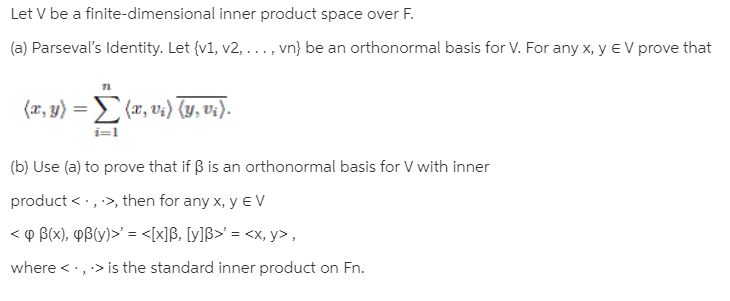# Let V be a finite-dimensional inner product space over F.(a) Parseval's Identity. Let {v1, v2, ..., vn} be an orthonormal basis for V. For any x, y e V prove that(π, 1) Σ(α, υ) U,υ.%3D(b) Use (a) to prove that if B is an orthonormal basis for V with innerproduct < ·, >, then for any x, y E V< o B(x), PB(Yy)>' = ' = ,where is the standard inner product on Fn.

Question
2 viewshelp_outlineImage TranscriptioncloseLet V be a finite-dimensional inner product space over F. (a) Parseval's Identity. Let {v1, v2, ..., vn} be an orthonormal basis for V. For any x, y e V prove that (π, 1) Σ(α, υ) U,υ. %3D (b) Use (a) to prove that if B is an orthonormal basis for V with inner product < ·, >, then for any x, y E V < o B(x), PB(Yy)>' = <[x]B, [y]B>' = , where <·,> is the standard inner product on Fn. fullscreen

### This question hasn't been answered yet.